# sbioparameterci

Compute confidence intervals for estimated parameters (requires Statistics and Machine Learning Toolbox)

## Syntax

``ci = sbioparameterci(fitResults)``
``ci = sbioparameterci(fitResults,Name,Value)``

## Description

example

````ci = sbioparameterci(fitResults)` computes 95% confidence intervals for the estimated parameters from `fitResults`, an `NLINResults object` or `OptimResults object` returned by the `sbiofit` function. `ci` is a `ParameterConfidenceInterval` object that contains the computed confidence intervals.```

example

````ci = sbioparameterci(fitResults,Name,Value)` uses additional options specified by one or more `Name,Value` pair arguments.```

## Examples

collapse all

Load the sample data to fit. The data is stored as a table with variables ID , Time , CentralConc , and PeripheralConc. This synthetic data represents the time course of plasma concentrations measured at eight different time points for both central and peripheral compartments after an infusion dose for three individuals.

```clear all load data10_32R.mat gData = groupedData(data); gData.Properties.VariableUnits = {'','hour','milligram/liter','milligram/liter'}; sbiotrellis(gData,'ID','Time',{'CentralConc','PeripheralConc'},'Marker','+',... 'LineStyle','none'); ```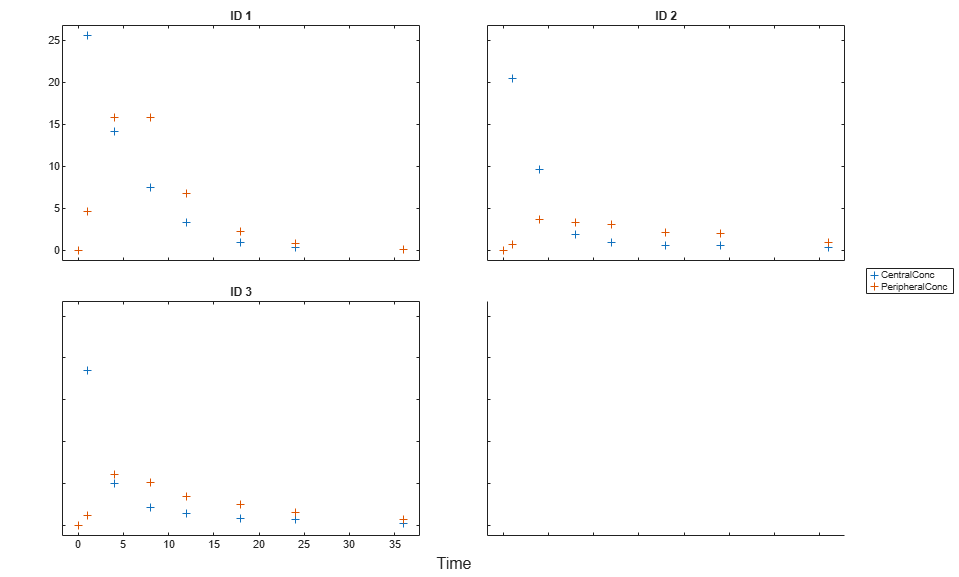Create Model

Create a two-compartment model.

```pkmd = PKModelDesign; pkc1 = addCompartment(pkmd,'Central'); pkc1.DosingType = 'Infusion'; pkc1.EliminationType = 'linear-clearance'; pkc1.HasResponseVariable = true; pkc2 = addCompartment(pkmd,'Peripheral'); model = construct(pkmd); configset = getconfigset(model); configset.CompileOptions.UnitConversion = true; ```

Define Dosing

Define the infusion dose.

```dose = sbiodose('dose','TargetName','Drug_Central'); dose.StartTime = 0; dose.Amount = 100; dose.Rate = 50; dose.AmountUnits = 'milligram'; dose.TimeUnits = 'hour'; dose.RateUnits = 'milligram/hour'; ```

Define Parameters

Define the parameters to estimate. Set the parameter bounds for each parameter. In addition to these explicit bounds, the parameter transformations (such as log, logit, or probit) impose implicit bounds.

```responseMap = {'Drug_Central = CentralConc','Drug_Peripheral = PeripheralConc'}; paramsToEstimate = {'log(Central)','log(Peripheral)','Q12','Cl_Central'}; estimatedParam = estimatedInfo(paramsToEstimate,... 'InitialValue',[1 1 1 1],... 'Bounds',[0.1 3;0.1 10;0 10;0.1 2]); ```

Fit Model

Perform an unpooled fit, that is, one set of estimated parameters for each patient.

```unpooledFit = sbiofit(model,gData,responseMap,estimatedParam,dose,'Pooled',false); ```

Perform a pooled fit, that is, one set of estimated parameters for all patients.

```pooledFit = sbiofit(model,gData,responseMap,estimatedParam,dose,'Pooled',true); ```

Compute Confidence Intervals for Estimated Parameters

Compute 95% confidence intervals for each estimated parameter in the unpooled fit.

```ciParamUnpooled = sbioparameterci(unpooledFit); ```

Display Results

Display the confidence intervals in a table format. For details about the meaning of each estimation status, see Parameter Confidence Interval Estimation Status.

```ci2table(ciParamUnpooled) ```
```ans = 12x7 table Group Name Estimate ConfidenceInterval Type Alpha Status _____ ______________ ________ __________________ ________ _____ ___________ 1 {'Central' } 1.422 1.1533 1.6906 Gaussian 0.05 estimable 1 {'Peripheral'} 1.5629 0.83143 2.3551 Gaussian 0.05 constrained 1 {'Q12' } 0.47159 0.20093 0.80247 Gaussian 0.05 constrained 1 {'Cl_Central'} 0.52898 0.44842 0.60955 Gaussian 0.05 estimable 2 {'Central' } 1.8322 1.7893 1.8751 Gaussian 0.05 success 2 {'Peripheral'} 5.3368 3.9133 6.7602 Gaussian 0.05 success 2 {'Q12' } 0.27641 0.2093 0.34351 Gaussian 0.05 success 2 {'Cl_Central'} 0.86034 0.80313 0.91755 Gaussian 0.05 success 3 {'Central' } 1.6657 1.5818 1.7497 Gaussian 0.05 success 3 {'Peripheral'} 5.5632 4.7557 6.3708 Gaussian 0.05 success 3 {'Q12' } 0.78361 0.65581 0.91142 Gaussian 0.05 success 3 {'Cl_Central'} 1.0233 0.96375 1.0828 Gaussian 0.05 success ```

Plot the confidence intervals. If the estimation status of a confidence interval is `success`, it is plotted in blue (the first default color). Otherwise, it is plotted in red (the second default color), which indicates that further investigation into the fitted parameters may be required. If the confidence interval is `not estimable`, then the function plots a red line with a centered cross. If there are any transformed parameters with estimated values 0 (for the log transform) and 1 or 0 (for the probit or logit transform), then no confidence intervals are plotted for those parameter estimates. To see the color order, type `get(groot,'defaultAxesColorOrder')`.

Groups are displayed from left to right in the same order that they appear in the `GroupNames` property of the object, which is used to label the x-axis. The y-labels are the transformed parameter names.

```plot(ciParamUnpooled) ```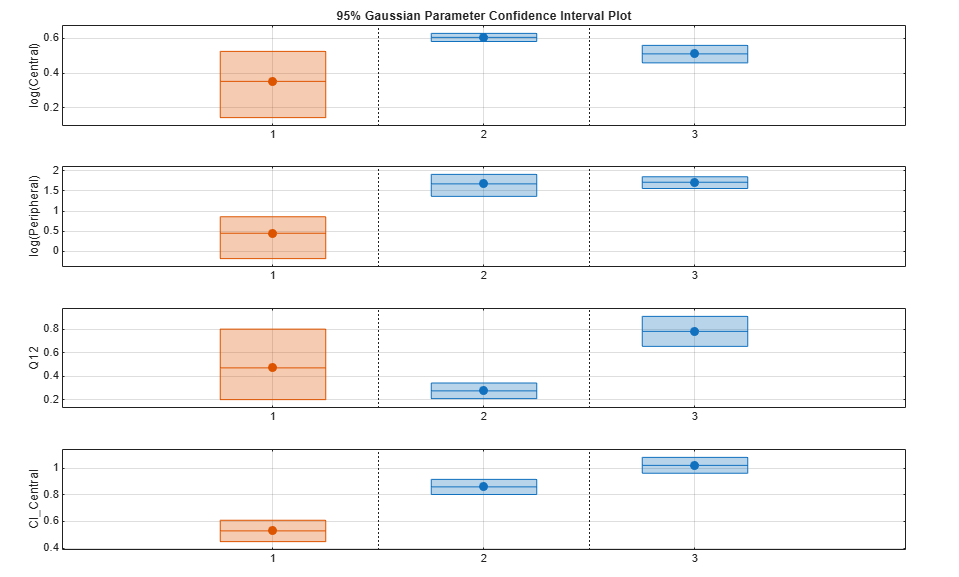Compute the confidence intervals for the pooled fit.

```ciParamPooled = sbioparameterci(pooledFit); ```

Display the confidence intervals.

```ci2table(ciParamPooled) ```
```ans = 4x7 table Group Name Estimate ConfidenceInterval Type Alpha Status ______ ______________ ________ __________________ ________ _____ ___________ pooled {'Central' } 1.6626 1.3287 1.9965 Gaussian 0.05 estimable pooled {'Peripheral'} 2.687 0.89848 4.8323 Gaussian 0.05 constrained pooled {'Q12' } 0.44956 0.11445 0.85152 Gaussian 0.05 constrained pooled {'Cl_Central'} 0.78493 0.59222 0.97764 Gaussian 0.05 estimable ```

Plot the confidence intervals. The group name is labeled as "pooled" to indicate such fit.

```plot(ciParamPooled) ```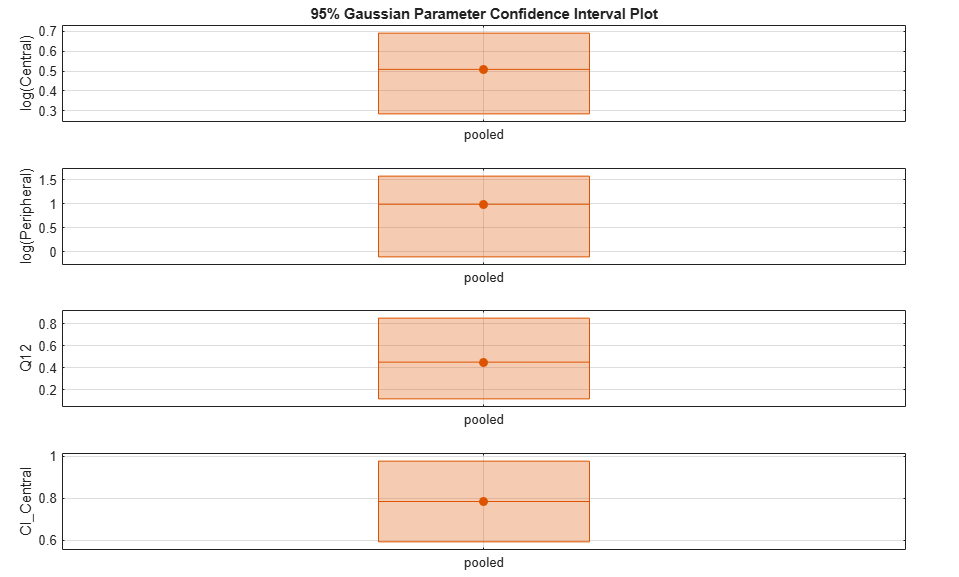Plot all the confidence interval results together. By default, the confidence interval for each parameter estimate is plotted on a separate axes. Vertical lines group confidence intervals of parameter estimates that were computed in a common fit.

```ciAll = [ciParamUnpooled;ciParamPooled]; plot(ciAll) ```You can also plot all confidence intervals in one axes grouped by parameter estimates using the 'Grouped' layout.

```plot(ciAll,'Layout','Grouped') ```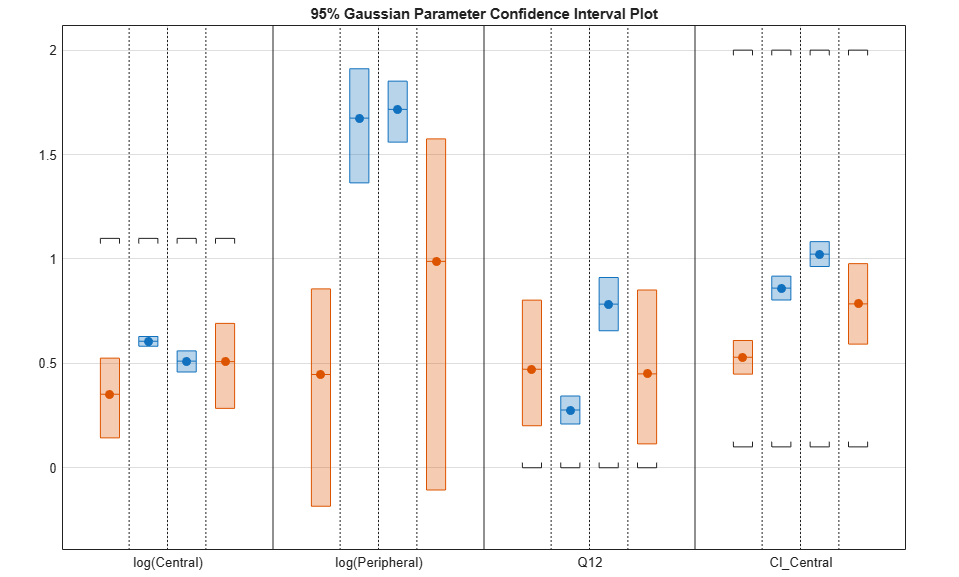In this layout, you can point to the center marker of each confidence interval to see the group name. Each estimated parameter is separated by a vertical black line. Vertical dotted lines group confidence intervals of parameter estimates that were computed in a common fit. Parameter bounds defined in the original fit are marked by square brackets. Note the different scales on the y-axis due to parameter transformations. For instance, the y-axis of `Q12` is in the linear scale, but that of `Central` is in the log scale due to its log transform.

Compute Confidence Intervals for Model Predictions

Calculate 95% confidence intervals for the model predictions, that is, simulation results using the estimated parameters.

```% For the pooled fit ciPredPooled = sbiopredictionci(pooledFit); % For the unpooled fit ciPredUnpooled = sbiopredictionci(unpooledFit); ```

Plot Confidence Intervals for Model Predictions

The confidence interval for each group is plotted in a separate column, and each response is plotted in a separate row. Confidence intervals limited by the bounds are plotted in red. Confidence intervals not limited by the bounds are plotted in blue.

```plot(ciPredPooled) ```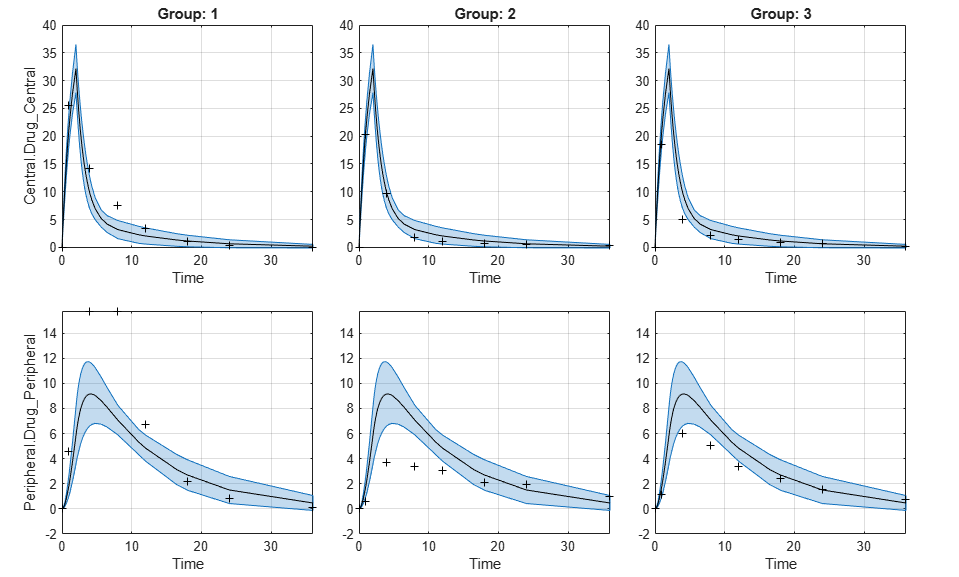```plot(ciPredUnpooled) ```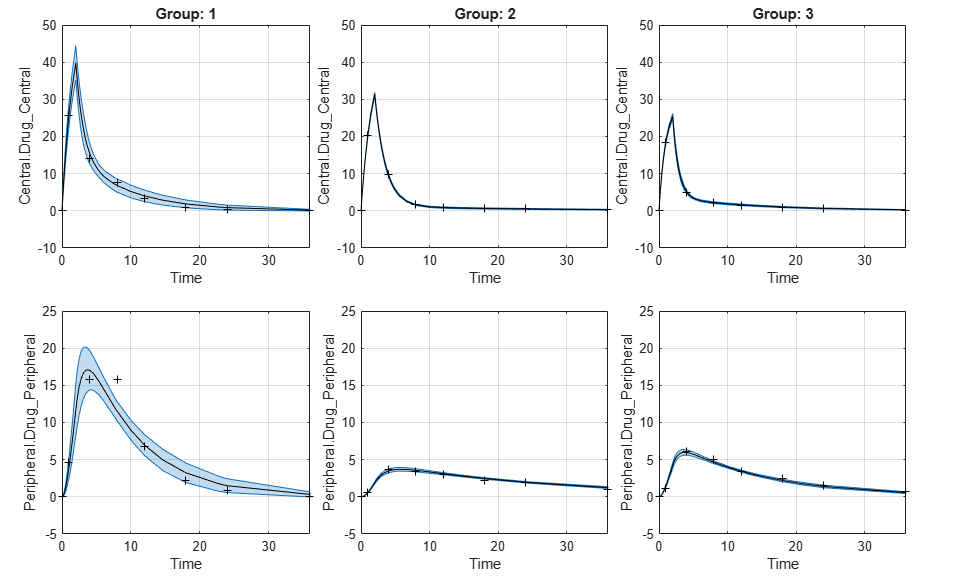## Input Arguments

collapse all

Parameter estimation results from `sbiofit`, specified as an `NLINResults object`, `OptimResults object`, or a vector of objects for unpooled fits that were returned from the same `sbiofit` call.

### Name-Value Pair Arguments

Specify optional comma-separated pairs of `Name,Value` arguments. `Name` is the argument name and `Value` is the corresponding value. `Name` must appear inside quotes. You can specify several name and value pair arguments in any order as `Name1,Value1,...,NameN,ValueN`.

Example: `'Alpha',0.01,'Type','profileLikelihood'` specifies to compute a 99% confidence interval using the profile likelihood approach.

Confidence level, `(1-Alpha) * 100%`, specified as the comma-separated pair consisting of `'Alpha'` and a positive scalar between 0 and 1. The default value is `0.05`, meaning a 95% confidence interval is computed.

Example: `'Alpha',0.01`

Confidence interval type, specified as the comma-separated pair consisting of `'Type'` and a character vector. The valid choices are:

• `'gaussian'`– Use the Gaussian approximation of the distribution of parameter estimates.

• `'profileLikelihood'`– Compute the profile likelihood intervals. This type is not supported for parameter estimates from hierarchical models, that is, estimated results from fitting different categories (such as Age or Sex). In other words, if you set the `CategoryVariableName` property of the `EstimatedInfo object` in your original fit, then the fit results are hierarchical, therefore, you cannot compute the `profileLikelihood` confidence intervals on the results. For details, see Profile Likelihood Confidence Interval Calculation.

• `'bootstrap'`– Compute confidence intervals using the bootstrap method.

Example: `'Type','bootstrap'`

Tolerance for the profile likelihood and bootstrap confidence interval computations, specified as the comma-separated pair consisting of `'Tolerance'` and a positive scalar.

The profile likelihood method uses this value as a termination tolerance. For details, see Profile Likelihood Confidence Interval Calculation.

The bootstrap method uses this value to determine whether a confidence interval is constrained by bounds specified in the original fit. For details, see Bootstrap Confidence Interval Calculation.

Example: `'Tolerance',1e-6`

Maximum step size used for computing profile likelihood curves, specified as the comma-separated pair consisting of `'MaxStepSize'` and a positive scalar, `[]`, or cell array. By default, this argument is set to `0.1`. If you set it to `[]`, then the maximum step size is set to 10% of the width of the Gaussian approximation of the confidence interval, if it exists. You can specify a maximum step size (or `[]`) for each estimated parameter using a cell array.

Example: `'MaxStepSize',0.5`

Number of samples for bootstrapping, specified as the comma-separated pair consisting of `'NumSamples'` and a positive integer. This number defines the number of fits that are performed during the confidence interval computation to generate bootstrap samples. The smaller the number is, the faster the computation of the confidence intervals becomes, at the cost of decreased accuracy.

Example: `'NumSamples',500`

Level of display returned to the command line, specified as the comma-separated pair consisting of `'Display'` and a character vector. `'off'` (default) or `'none'` displays no output. `'final'` displays a message when a computation finishes. `'iter'` displays output at each iteration.

Example: `'Display','final'`

Logical flag to compute confidence intervals in parallel, specified as the comma-separated pair consisting of `'UseParallel'` and `true` or `false`. By default, the parallel options in the original fit are used. If this argument is set to `true` and Parallel Computing Toolbox™ is available, the parallel options in the original fit are ignored, and confidence intervals are computed in parallel.

For the Gaussian confidence intervals:

• If the input `fitResults` is a vector of results objects, then the computation of confidence intervals for each object is performed in parallel. The Gaussian confidence intervals are quick to compute. So, it might be more beneficial to parallelize the original fit (`sbiofit`) and not set `UseParallel` to true for `sbioparameterci`.

For the Profile Likelihood confidence intervals:

• If the number of results objects in the input `fitResults` vector is greater than the number of estimated parameters, then the computation of confidence intervals for each object is performed in parallel.

• Otherwise, the confidence intervals for all estimated parameters within one results object are computed in parallel before the function moves on to the next results object.

For the Bootstrap confidence intervals:

• The function forwards the `UseParallel` flag to `bootci`. There is no parallelization over the input vector of results objects.

### Note

If you have a global stream for random number generation with several substreams to compute in parallel in a reproducible fashion, `sbioparameterci` first checks to see if the number of workers is same as the number of substreams. If so, `sbioparameterci` sets `UseSubstreams` to `true` in the `statset` option and passes it to `bootci`. Otherwise, the substreams are ignored by default.

Example: `'UseParallel',true`

## Output Arguments

collapse all

Confidence interval results, returned as a `ParameterConfidenceInterval` object. For an unpooled fit, `ci` can be a vector of `ParameterConfidenceInterval` objects.

collapse all

### Gaussian Confidence Interval Calculation

The function uses the Wald test statistic  to compute the confidence intervals. Assuming that there are enough data, the parameter estimates, Pest, are approximately Student's t-distributed with the covariance matrix S (the `CovarianceMatrix` property of the results object) returned by `sbiofit`.

The confidence interval for the ith parameter estimate Pest,i is computed as follows:

$Pest,i±\sqrt{Si,i}*Tinv\left(1-\frac{Alpha}{2}\right)$, where Tinv is the Student's t inverse cumulative distribution function (`tinv`) with the probability `1-(Alpha/2)`, and Si,i is the diagonal element (variance) of the covariance matrix S.

In cases where the confidence interval is constrained by the parameter bounds defined in the original fit, the confidence interval bounds are adjusted according to the approach described by Wu, H. and Neale, M. .

#### Setting Estimation Status

• For each parameter estimate, the function first decides whether the confidence interval of the parameter estimate is unbounded. If so, the function sets the estimation status of the corresponding parameter estimate to `not estimable`.

• Otherwise, if the confidence interval for a parameter estimate is constrained by a parameter bound defined in the original fit, the function sets the estimation status to `constrained`. Parameter transformations (such as `log`, `probit`, or `logit`) impose implicit bounds on the estimated parameters, for example, positivity constraints. Such bounds can lead to the overestimation of confidence, that is, the confidence interval can be smaller than expected.

• If no confidence interval has the estimation status ```not estimable``` or `constrained`, then the function sets the estimation statuses of all parameter estimates to `success`. Otherwise, the estimation statuses of remaining parameter estimates are set to `estimable`.

### Profile Likelihood Confidence Interval Calculation

Define L to be the likelihood, LH, of the parameter estimates (stored in the `ParameterEstimates` property of the results object) returned by `sbiofit`, $L=LH\left(Pest\right)$, where Pest is a vector of parameter estimates, Pest,1, Pest,2, …, Pest,n.

The profile likelihood function PL for a parameter Pi is defined as:, where n is the total number of parameters.

Per Wilks's Theorem , the likelihood ratio test statistic,, is chi-square distributed with 1 degree of freedom.

Therefore, find all Pi so that:.

Equivalently,, whereis the target value used in computing the log profile likelihood curve as described next.

#### Computing Log Profile Likelihood Curve

1. Start at Pest,i and evaluate the likelihood L.

2. Compute the log profile likelihood at Pest,i `+ k * MaxStepSize` for each side (or direction) of the confidence interval, that is, `k = 1, 2, 3,…` and ```k = -1, -2, -3,…```.

3. Stop if one of these stopping criteria is met on each side.

• The log profile likelihood falls below the target value. In this case, start bisecting between Pbelow and Pabove, where Pbelow is the parameter value with the largest log profile likelihood value below the target value, and Pabove the parameter value with the smallest log profile likelihood value greater than the target value. Stop the bisection if one of the following is true:

• Either neighboring log profile likelihood values are less than Tolerance apart. Set the status for the corresponding side of the confidence interval to `success`.

• The bisection interval becomes smaller than `max(Tolerance,2*eps('double'))` and the profile likelihood curve computed so far is above the target value. Set the status of the corresponding side to ```not estimable```.

• The linear gradient approximation of the profile likelihood curve (finite difference between two neighboring parameter values) is larger than -Tolerance (the negative value of the tolerance). Set the status of the corresponding side to `not estimable`.

• The step is limited by a bound defined in the original fit. Evaluate at the bound and set the status of the corresponding side to `constrained`.

#### Setting Estimation Status

• If both sides of the confidence interval are unsuccessful, that is, have the status `not estimable`, the function sets the estimation status (`ci`.Results.Status) to `not estimable`.

• If no side has the status `not estimable` and one side has the status `constrained`, the function sets the estimation status (`ci`.Results.Status) to `constrained`.

• If the computation for all parameters on both sides of the confidence intervals is successful, set the estimation status (`ci`.Results.Status) to `success`.

• Otherwise, the function sets the estimation statuses of the remaining parameter estimates to `estimable`.

### Bootstrap Confidence Interval Calculation

The `bootci` function from Statistics and Machine Learning Toolbox™ is used to compute the bootstrap confidence intervals. The first input nboot is the number of samples (`NumSamples`), and the second input bootfun is a function that performs these actions:

• Resample the data (independently within each group, if multiple groups are available).

• Run a parameter fit with the resampled data.

• Return the estimated parameters.

#### Setting Estimation Status

If a confidence interval is closer than `Tolerance` to a parameter bound, as defined in the original fit, the function sets the estimation status to `constrained`. If all confidence intervals are further away from the parameter bounds than `Tolerance`, the function sets the status to `success`. Otherwise, it is set to `estimable`.

 Wald, A. "Tests of Statistical Hypotheses Concerning Several Parameters when the Number of Observations is Large." Transactions of the American Mathematical Society. 54 (3), 1943, pp. 426-482.

 Wu, H., and M.C. Neale. "Adjusted Confidence Intervals for a Bounded Parameter." Behavior Genetics. 42 (6), 2012, pp. 886-898.

 Wilks, S.S. "The Large-Sample Distribution of the Likelihood Ratio for Testing Composite Hypotheses." The Annals of Mathematical Statistics. 9 (1), 1938, pp. 60–62.

##### SupportGet trial now